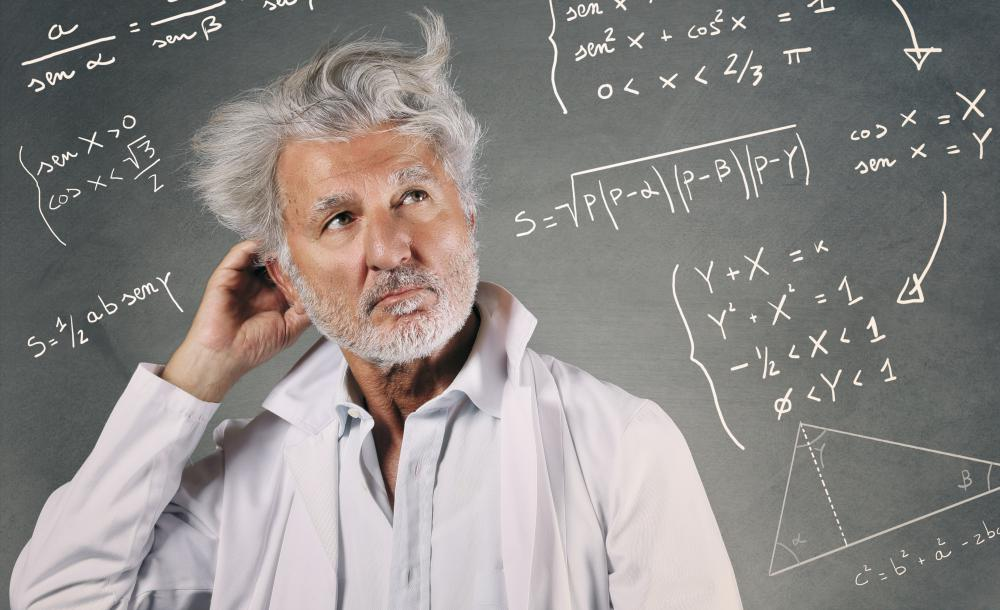# What Is a Coset?

David Isaac Rudel

A coset is a specific type of subset of a mathematical group. For example, one might consider the set of all integral multiples of 7, {... -14, -7, 0, 7, 14 ...}, which can be denoted as 7Z. Adding 3 to each number generates the set {... -11, -4, 3, 10, 17 ...}, which mathematicians describe as 7Z + 3. This latter set is called the coset of 7Z generated by 3.A coset is a specific type of subset of a mathematical group.

There are two important properties of 7Z. If a number is a multiple of 7, so is its additive inverse. The additive inverse of 7 is -7, the additive inverse of 14 is -14, and so on. Also, adding a multiple of 7 to another multiple of 7 yields a multiple of 7. Mathematicians describe this by saying that the multiples of 7 are “closed” under the operation of addition.

These two characteristics are why 7Z is called a subgroup of the integers under addition. Only subgroups have cosets. The set of all cubic numbers, {... -27, -8, -1, 0, 1, 8, 27 ...}, does not have cosets in the same way as 7Z because it is not closed under addition: 1 + 8 = 9, and 9 is not a cubic number. Similarly, the set of all positive even numbers, {2, 4, 6, ...}, does not have cosets because it does not contain inverses.

The reason for these stipulations is that every number should be in exactly one coset. In the case of {2, 4, 6, ...}, 6 is in the coset generated by 4 and is in the coset generated by 2, but those two cosets are not identical. These two criteria suffice to ensure that each element is in exactly one coset.

Cosets exist in any group, and some groups are far more complicated than the integers. A useful group that one might consider is the set of all the ways to move a square without changing the region it covers. If a square is rotated 90 degrees, there is no apparent change in the shape. Similarly, it can be flipped vertically, horizontally or across either diagonal without changing the region the square covers. Mathematicians call this group D4.

D4 has eight elements. Two elements are considered identical if they leave all the corners in the same place, so rotating the square clockwise four times is considered the same as doing nothing. With this in mind, the eight elements can be denoted e, r, r2, r3, v, h, dd, and dd. The “e” refers to doing nothing, and “r2” denotes doing two rotations. Each of the last four elements refers to flipping the square: vertically, horizontally or along its upward- or downward-slanting diagonals.

The integers are an Abelian group, meaning its operation satisfies the commutative law: 3 + 2 = 2 + 3. D4 is not Abelian. Rotating a square and then flipping it horizontally does not move the corners in the same way as flipping it and then rotating it.

When working in non-commutative groups, mathematicians typically use a * to describe the operation. A little work shows that rotating the square and then flipping it horizontally, r * h, is the same as flipping it across its downward diagonal. Thus r * h = dd. Flipping the square and then rotating it is equivalent to flipping it across its upward diagonal, so r * h = du.

Order matters in D4, so one must be more precise when describing cosets. When working in the integers, the phrase “the coset of 7Z generated by 3” is unambiguous because it does not matter whether 3 is added on the left or right of each multiple of 7. For a subgroup of D4, however, different orders will create different cosets. Based on the calculations describe earlier, r*H, the left coset of H generated by r—equals {r, dd} but H*r equals (r, du}. The requirement that no element be in two different cosets does not apply when comparing right cosets to left cosets.

The right cosets of H do not match its left cosets. Not all subgroups of D4 share this property. One can consider the subgroup R of all rotations of the square, R={e, r, r2, r3}.

A little calculation shows that its left cosets are the same as its right cosets. Such a subgroup is called a normal subgroup. Normal subgroups are extremely important in abstract algebra because they always encode extra information. For example, the two possible cosets of R equate to the two possible situations “the square has been flipped” and “the square has not been flipped.”

## You might also Like